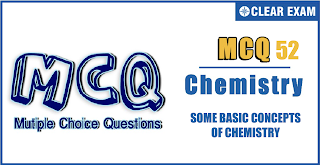## SOME BASIC CONCEPTS OF CHEMISTRY Quiz-52

IIT JEE exam which consists of JEE Main and JEE Advanced is one of the most important entrance exams for engineering aspirants. The exam is held for candidates who are aspiring to pursue a career in the field of engineering and technical studies. Chemistry is important because everything you do is chemistry! Even your body is made of chemicals. Chemical reactions occur when you breathe, eat, or just sit there reading. All matter is made of chemicals, so the importance of chemistry is that it's the study of everything..

•  A
•  B
•  C
•  D
•  A
•  B
•  C
•  D
Solution

(c) AgNO_3+NaCl→AgCl+NaNO_3 1 mol 1 mol 1 mol 170 g 58.5 g 143.5 g 1.70 g AgNO_3 gives 1.435 g AgCl 5.85 g NaCl gives 14.35 g AgCl Thus, AgNO_3 is the limiting reactant AgCl formed =1.435 g

•  A
•  B
•  C
•  D
Solution
(d) 1 mol _6^12 C=12g 1 amu=1/N_0 Number of moles in amu=1/(12N_0 )

Q4.

According to Dalton’s atomic theory, the smallest particle in which matter can exist, is called
•  An Atom
•  An Ion
•  An Electron
•  A Molecule
Solution
(a) By Dalton’s theory, atom is the smallest particle

Q5.Which has the maximum percentage of chlorine?
•  C6H6CL6
•  C6H5CL
•  CH3CL
•  CCL4
Solution
(d) (a) Cl%=35.5/(35.5+12+1)=35.5/48.5=x/y (b)=35.5/(72+5+35.5)=35.5/112.5 (c)=35.5/(15+35.5)=35.5/50.5 (d)=(35.5×4)/(12+35.5×4)=35.5/(3+35.5)=35.5/38.5 In all cases value of X=35.5 Smaller the value of Y, larger the percentage of Cl

Q6. The number of moles of KMnO_4 that will be needed to react with 1 mol of sulphite ion in acidic solution is
•  2/5
•  3/5
•  4/5
•  1
Solution
(a) SO_3^( 2-)→SO_4^( 2-)+2e^- (n=2) 1/5 mol=1/2 mol 2/5 mol=1 mol

•  A
•  B
•  C
•  D
Solution
(b) 2N_2+ 3O_2→2N_2 O_3,(% yield=80%) Initial mol of N_2=7.0/28=0.25 mol Moles of N_2 converted = 0.25×80/100=0.2 2 mol N_2=3 mol O_2 0.2 mol N_2 = 0.3 mol O_2 (1 mol O_2 = 2 oxygen atom) =2×0.3 mol O atom = 2×0.3×6.02×10^23=3.6×10^23

Q8.The amount of zinc (atomic weight = 65) necessary to produce 224 mL of H_2 by the reaction with an acid will be
•  0.65g
•  6.5g
•  0.065g
•  65g
Solution
(a) Zn+2HCl→ZnCl_2+H_2 65 g 22400 mL 0.65 g 224 mL

Q9.What volume of 0.1 M Ba(OH)_2 will be required to neutralise a mixture of 50 mL of 0.1 M HCl and 100 mL of 0.2 M H_3 PO_4 using methyl red indicator?
•  25 mL
•  50 mL
•  100 mL
•  125 mL
Solution
(d) Methyl red indicates complete ionisation of HCl (n=1) and first step ionisation of H_3 PO_4 (n=1) First case: Ba(OH)_2≡HCl N_1 V_1=N_2 V_2 0.1×2×V_1=0.1×1×50 V_1=25 mL Second case: (Ba(OH)_2≡H_3 PO_4 (n=1) 0.1×2×V_1=0.2×1×100 Total volume =100+25=125 mL

Q10.Suppose elements X and Y combine to form two compounds XY_2 and X_3 Y_2 when 0.1 mole of former weigh 10 g while 0.05 mole of the latter weigh 9 g. What are the atomic weights of X and Y
•  40,30
•  60,40
•  20,30
•  30,20
Solution
(a) i. X + 2Y→XY_2 0.1 mol of XY_2 = 10 g 1 mol of XY_2 = 100 g ii. 3X+ 2Y→ X_3 Y_2 0.05 mol of X_3 Y_2=9g 1 mol of X_3 Y_2=9/0.05=180g ├ ■(∴X+2Y=10@3X+2Y=180)] ■(Mw XY_2=100@Mw X_2 Y_3=180) Solve for X and Y X = 40 g Y = 30 g#### Written by: AUTHORNAME

AUTHORDESCRIPTION## Want to know more

Please fill in the details below:

## Latest NEET Articles\$type=three\$c=3\$author=hide\$comment=hide\$rm=hide\$date=hide\$snippet=hide

Name

ltr
item
BEST NEET COACHING CENTER | BEST IIT JEE COACHING INSTITUTE | BEST NEET & IIT JEE COACHING: SOME BASIC CONCEPTS OF CHEMISTRY Quiz-52
SOME BASIC CONCEPTS OF CHEMISTRY Quiz-52
https://1.bp.blogspot.com/-t7vN1pczIm4/YOXR2S1NprI/AAAAAAAAUQ8/L0TA8QTxEKEUMd9fVLM9WJ-98uzP6sirgCLcBGAsYHQ/s320/Screenshot%2B2021-07-06%2Bat%2B6.35.04%2BPM.png
https://1.bp.blogspot.com/-t7vN1pczIm4/YOXR2S1NprI/AAAAAAAAUQ8/L0TA8QTxEKEUMd9fVLM9WJ-98uzP6sirgCLcBGAsYHQ/s72-c/Screenshot%2B2021-07-06%2Bat%2B6.35.04%2BPM.png
BEST NEET COACHING CENTER | BEST IIT JEE COACHING INSTITUTE | BEST NEET & IIT JEE COACHING
https://www.cleariitmedical.com/2021/07/some-basic-concepts-of-chemistry-quiz-52.html
https://www.cleariitmedical.com/
https://www.cleariitmedical.com/
https://www.cleariitmedical.com/2021/07/some-basic-concepts-of-chemistry-quiz-52.html
true
7783647550433378923
UTF-8

STAY CONNECTED